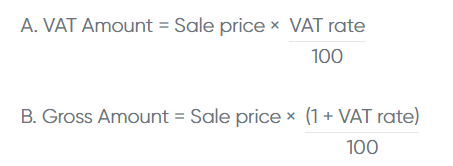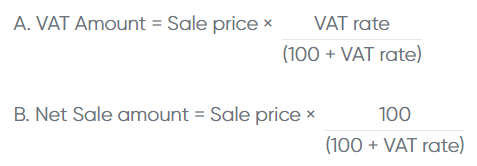# How to calculate VAT in UAE?

The Federal Tax Authority (FTA) is implementing the Value Added Tax (VAT) in United Arab Emirates (UAE) w.e.f 1st January 2018. The standard VAT rate in UAE is 5%.

Every VAT-registered business in UAE must pay VAT while purchasing goods or services and collect VAT while selling them. So, calculating VAT is a daily activity for any type of business.

This article takes you through the VAT calculation formula, input VAT, output VAT, VAT calculation example, FAQs etc.

## What is VAT?

VAT falls under indirect taxation and is imposed on the sale and import of goods and services, with certain exceptions. More than 160 countries around the world are implementing the VAT system.

VAT is paid and collected by the buyer and seller, respectively, at every stage of the supply chain. Suppose, in the case of a manufacturer, from purchasing raw materials by the manufacturer until a retailer sells the end product to a consumer.

The United Arab Emirates (UAE) government introduced VAT w.e.f 1st January 2018 and started charging 5% for taxable sales and imports.

## VAT calculation formula

There are two methods to do VAT calculation in UAE. In the first one, you must add VAT to the sale price; in the second one, you must exclude the VAT from the sale price. Let’s see one by one:

1. Adding VAT to the sale price: This method is used when VAT is not included in the sale price. Below are the formulas to add the VAT to the sale price:1. Excluding VAT from sale price: This method is used when VAT is included in the sale price. You can use the below formulas to exclude VAT from the sale price:## VAT calculation example

Let’s understand VAT calculation using an example.

Sale amount = AED 1,000

VAT rate = 5%

1. When the sale price is exclusive of VAT:
1. VAT amount = AED 1,000 x  (5/100)   = AED 50
2. Gross amount = AED 1,000 x (1+ (5/100)) = AED 1,050
2. When the sale price is inclusive of VAT:
1. VAT amount = AED 1,000 x (5/(100+5))  = AED 47.62
2. Net sale amount =  AED 1,000 x (100/ (100+5)) = AED 952.38

## What are input VAT and output VAT?

The VAT system is largely based on the input VAT and output VAT. Let’s understand these with examples.

### Input VAT

The tax paid on purchasing goods or services is called input VAT.

Suppose Mr Rashid purchased goods worth AED  5,000 + 5% VAT from Sultan Distributors. The tax of 250 paid by Mr Rashid is an input VAT.

### Output VAT

The tax collected while selling goods or services is called output VAT.

Suppose Mansour sold goods worth 10,000 with 5% VAT to Raheem Enterprises. The VAT of 500 collected by Mansour is an output vat.

## How to calculate VAT payment?

VAT-registered taxpayers can pay VAT after deducting input VAT (paid on purchases) from the output VAT (collected on sales). You can arrive at the net VAT payable using the below formula:

Net VAT payment = Output VAT – Input VAT

You need to record the total output VAT collected and input VAT paid during the tax period. You can apply the above formula once you have calculated the output and input VAT.

If your output VAT exceeds the input VAT, the difference will be the VAT payable. Suppose the output VAT is less than the Input VAT; you don’t need to pay tax, and will get the refund.

## FAQs

#### How is the VAT calculated in UAE?

You should add a 5% VAT to the sale price. For example, the taxable products/services price is AED 100; the buyer has to pay the VAT of AED 5 (AED 100 x 5%).

#### What is the VAT rate in UAE?

The standard VAT rate is 5% in UAE. However, the government categorised a few goods and services under exempt and zero-rated supplies where no tax is charged.

#### How do you add 5% VAT on a calculator?

You must input the sale price and multiply it with the (1+ VAT rate) to obtain the gross amount to be collected.

#### How do you exclude VAT from the price of a product?

You’ve to exclude the VAT from the sale price using the second method mentioned above.

#### What is the VAT calculation Formula to calculate VAT in UAE?

VAT = Price inclusive of tax x (VAT rate ÷ (100 + VAT rate))

#### What is the VAT on 100?

For instance, if the price of the product is AED 100, in that case, the Input VAT will be 100x5%= AED 5.00.

CONTENTS
ClearTax is a product by Defmacro Software Pvt. Ltd.